Math Olympiad Test: Number System- 2

# Math Olympiad Test: Number System- 2

Test Description

## 15 Questions MCQ Test Mathematics Olympiad for Class 9 | Math Olympiad Test: Number System- 2

Math Olympiad Test: Number System- 2 for Class 9 2022 is part of Mathematics Olympiad for Class 9 preparation. The Math Olympiad Test: Number System- 2 questions and answers have been prepared according to the Class 9 exam syllabus.The Math Olympiad Test: Number System- 2 MCQs are made for Class 9 2022 Exam. Find important definitions, questions, notes, meanings, examples, exercises, MCQs and online tests for Math Olympiad Test: Number System- 2 below.
Solutions of Math Olympiad Test: Number System- 2 questions in English are available as part of our Mathematics Olympiad for Class 9 for Class 9 & Math Olympiad Test: Number System- 2 solutions in Hindi for Mathematics Olympiad for Class 9 course. Download more important topics, notes, lectures and mock test series for Class 9 Exam by signing up for free. Attempt Math Olympiad Test: Number System- 2 | 15 questions in 15 minutes | Mock test for Class 9 preparation | Free important questions MCQ to study Mathematics Olympiad for Class 9 for Class 9 Exam | Download free PDF with solutions
 1 Crore+ students have signed up on EduRev. Have you?
Math Olympiad Test: Number System- 2 - Question 1

### Which of the following number is irrational?

Detailed Solution for Math Olympiad Test: Number System- 2 - Question 1

Since,
- = 0.875 (Terminating decimal)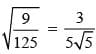(Irrational)
93/300 = 31/100 = 0.31 (Terminating decimal)
190/30 =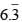(Repeating decimal)
∵ Repeating and terminating decimals are rational numbers.

Math Olympiad Test: Number System- 2 - Question 2

### (243/32)-0.8 then the value of ‘t’ will be

Detailed Solution for Math Olympiad Test: Number System- 2 - Question 2

Here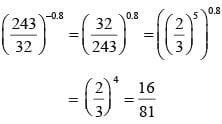Math Olympiad Test: Number System- 2 - Question 3

### If m – n = 1, then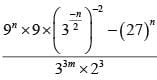= (1/3)x then x =

Detailed Solution for Math Olympiad Test: Number System- 2 - Question 3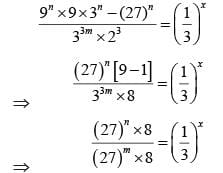⇒ (3)3(n-m) = (3)-x
⇒ x = 3(m – n) = 3 [m - n = 1]

Math Olympiad Test: Number System- 2 - Question 4

If √3 = 1.732 and √5 = 2.236 then the value of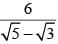is

Detailed Solution for Math Olympiad Test: Number System- 2 - Question 4

We have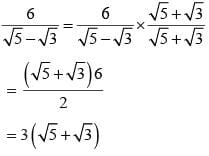= 3(2.236+1.732)
= 3(3.968)
= 11.904

Math Olympiad Test: Number System- 2 - Question 5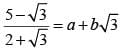then the respective values of  α and b are

Detailed Solution for Math Olympiad Test: Number System- 2 - Question 5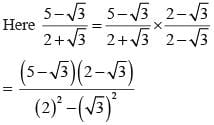= 10 + 3 – 7√3 = α + b
= 13 – 7√3 = α + b√3
⇒ α = 13, b = –7

Math Olympiad Test: Number System- 2 - Question 6

If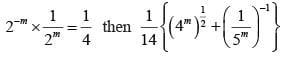is equal to

Detailed Solution for Math Olympiad Test: Number System- 2 - Question 6

2-m x 2-m =2-2
⇒ (2)-2m = (2)-2
⇒ m = 1
Substituting the value of m in,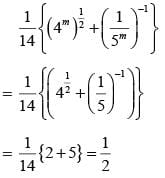Math Olympiad Test: Number System- 2 - Question 7

The value of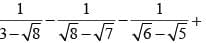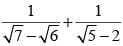is

Detailed Solution for Math Olympiad Test: Number System- 2 - Question 7

Here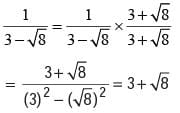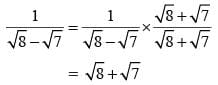Similarly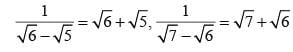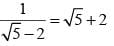Rearranging all the terms in the required pattern, we have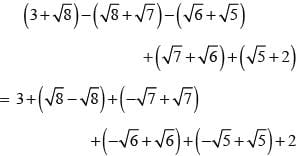= 3 + 2 = 5

Math Olympiad Test: Number System- 2 - Question 8

10x = 64, then the value of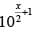is

Detailed Solution for Math Olympiad Test: Number System- 2 - Question 8

∵ 10x = 64 ⇒ (10x)1/2 = (64)1/2 = 8
∴ 10x/2 = 8 ⇒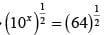= 8 x 10 = 80

Math Olympiad Test: Number System- 2 - Question 9

If  x-2 = 64, then x0 + x1/3

Detailed Solution for Math Olympiad Test: Number System- 2 - Question 9

∵ x–2 = 64
⇒ x-1 = 8
⇒ x = 1/8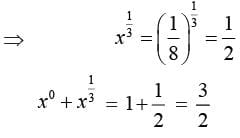Math Olympiad Test: Number System- 2 - Question 10

If x = 1– √2, then the value of(x - 1/x)3 is

Detailed Solution for Math Olympiad Test: Number System- 2 - Question 10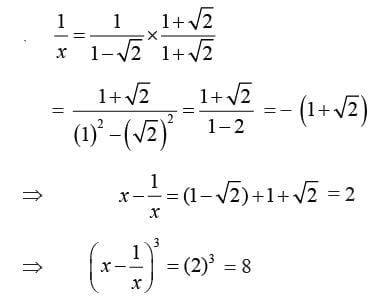Math Olympiad Test: Number System- 2 - Question 11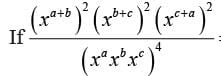= y then y is equal to

Detailed Solution for Math Olympiad Test: Number System- 2 - Question 11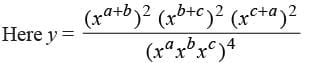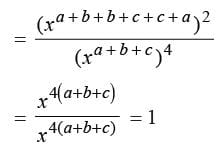Math Olympiad Test: Number System- 2 - Question 12

If x = 2 + √3, then x + 1/x is equal to:

Detailed Solution for Math Olympiad Test: Number System- 2 - Question 12

Given x = 2 + √3 then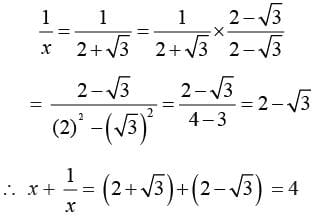Math Olympiad Test: Number System- 2 - Question 13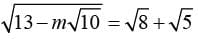, then m =

Detailed Solution for Math Olympiad Test: Number System- 2 - Question 13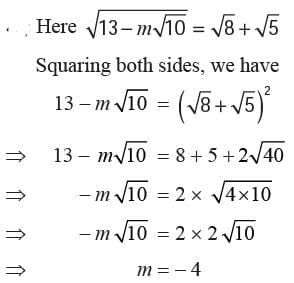Math Olympiad Test: Number System- 2 - Question 14

If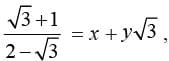then x, y have values equal to

Detailed Solution for Math Olympiad Test: Number System- 2 - Question 14

Rationalising the denominator we have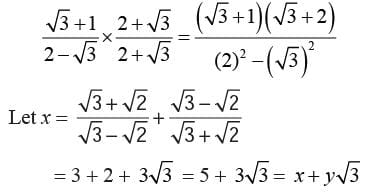∴  x = 5, y = 3

Math Olympiad Test: Number System- 2 - Question 15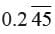in the simplest form will be equal to:

Detailed Solution for Math Olympiad Test: Number System- 2 - Question 15

Given n =then x = 0.24545
10x = 2.4545 ...(i)
and 100x = 24.54545
and 1000x = 245.454545 ...(iii)
Subtracting eq (i) and eq (iii), we get
990x = 243
⇒ x = 243/990 = 27/110

## Mathematics Olympiad for Class 9

1 videos|43 tests
 Use Code STAYHOME200 and get INR 200 additional OFF Use Coupon Code
Information about Math Olympiad Test: Number System- 2 Page
In this test you can find the Exam questions for Math Olympiad Test: Number System- 2 solved & explained in the simplest way possible. Besides giving Questions and answers for Math Olympiad Test: Number System- 2, EduRev gives you an ample number of Online tests for practice

## Mathematics Olympiad for Class 9

1 videos|43 tests